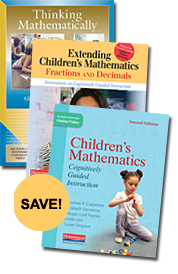# Cognitively Guided Instruction Bundle

By Thomas P Carpenter, Elizabeth Fennema, Megan Loef Franke, Linda Levi, Susan B. Empson

Multiply your understanding of children’s mathematical thinking and save money by purchasing the definitive collection of books about Cognitively Guided Instruction. In Children’s Mathematics, Second Edition, Extending Children’s Mathematics, and Thinking Mathematically, the authors detail:

• how children solve problems using their intuitive understanding of addition, subtraction, multiplication, division, and base-ten number concepts
• instruction that promotes children’s engagement in essential mathematical practices
• how teachers can recognize and support children’s use of properties of operations and other algebraic concepts in a manner that deepens their understanding and provides a solid foundation for learning algebra
• strategies to build meaning for fractions and decimals through discussing and solving word problems.
...

### Bundle

In Stock

Web/School Price: \$77.10

#### Full Description

Multiply your understanding of children’s mathematical thinking and save money by purchasing the definitive collection of books about Cognitively Guided Instruction. In Children’s Mathematics, Second Edition, Extending Children’s Mathematics, and Thinking Mathematically, the authors detail:

• how children solve problems using their intuitive understanding of addition, subtraction, multiplication, division, and base-ten number concepts
• instruction that promotes children’s engagement in essential mathematical practices
• how teachers can recognize and support children’s use of properties of operations and other algebraic concepts in a manner that deepens their understanding and provides a solid foundation for learning algebra
• strategies to build meaning for fractions and decimals through discussing and solving word problems.

These books provide classroom examples, sample problems, teacher reflections, and an extensive collection of video clips that will give you the resources you need to support the development of deep mathematical thinking in your students.